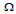# Digital Electronics - Multivibrators and 555 Timer

### Exercise :: Multivibrators and 555 Timer - General Questions

26.

What is the pulse width of the output of a 74123 if an external 4.7-kresistor and a 0.022-F capacitor are used?

 A. 290 ms B. 29 ms C. 33.3s D. 290s

Explanation:

No answer description available for this question. Let us discuss.

27.

To obtain a 50% duty cycle in an astable 555 timer circuit:

 A. tLO = tHI B. RA = RB and short RB with a diode during the capacitor charging cycle C. capacitor voltage must rise above 1/3 VCC D. tLO = tHI, RA = RB, and short RB with a diode during the capacitor charging cycle

Explanation:

No answer description available for this question. Let us discuss.

28.

What is another name for a bistable multivibrator?

 A. an on-off switch B. an oscillator C. a flip-flop

Explanation:

No answer description available for this question. Let us discuss.

29.

An astable 555 timer has the following number of stable states:

 A. 0 B. 1 C. 2 D. 3

Explanation:

No answer description available for this question. Let us discuss.

30.

Which mode of operation is being used when a 555 timer chip has two external resistors and an external capacitor?

 A. monostable B. pulse stretching C. Schmitt triggering D. astable